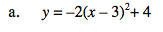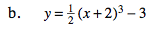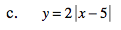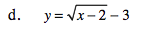Home > INT3 > Chapter 7 > Lesson 7.2.4 > Problem7-98

7-98.
1. Use your knowledge of transforming parent graphs to sketch a graph of each function below. Homework Help ✎

1. y = –2(x – 3)2 + 4

2. y =(x + 2)3 – 3

3. y = 2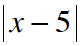4. y =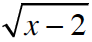– 3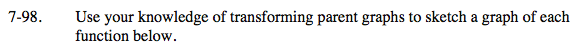Refer to the Math Notes box in Lesson 2.2.5. Use a graphing calculator to check your answers. If you need more help, review the Checkpoint 5 materials.